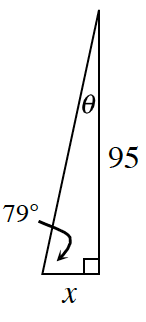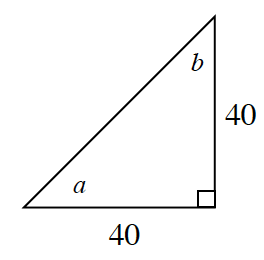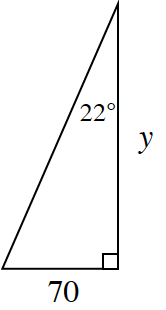### Home > CCG > Chapter 4 > Lesson 4.1.2 > Problem4-17

4-17.

Use your Trig Table Toolkit from problem 4-16 to help you find the value of each variable below.

Review the Math Notes box in this lesson.

1.Triangle Angle Sum Theorem, $79º+θ+90º=180º$

$θ=11º$

$\frac{95}{x}\approx \frac{5}{1}$

$x\approx18.46$

1.Notice that the legs of the right triangle are the same lengths.

1.Look at the hints in part (a).